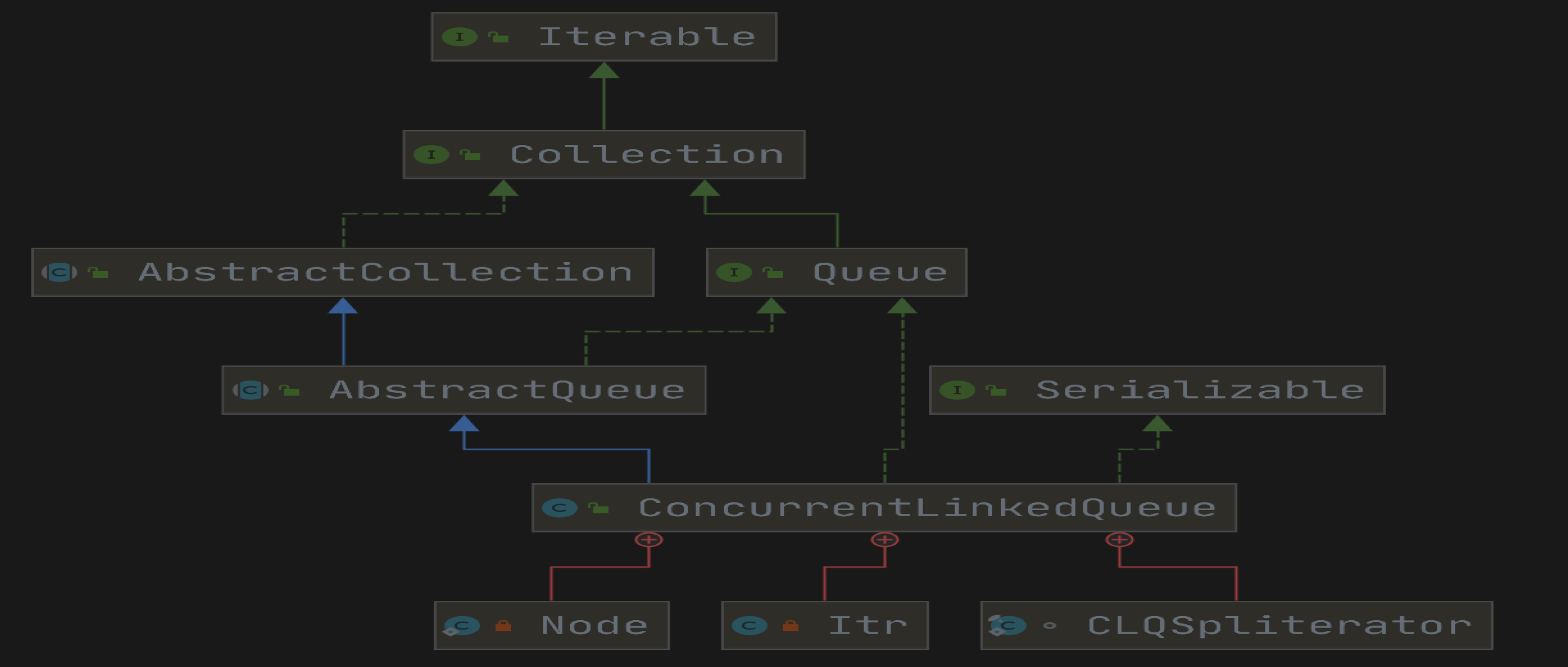### 介绍

#### 基本使用

public class ConcurrentLinkedQueueTest {    public static void main(String[] args) {        ConcurrentLinkedQueue<String> queue = new ConcurrentLinkedQueue<String>();        // 将指定元素插入此队列的尾部。        queue.add("liuzhihang");        // 将指定元素插入此队列的尾部。        queue.offer("liuzhihang");        // 获取但不移除此队列的头，队列为空返回 null。        queue.peek();        // 获取并移除此队列的头，此队列为空返回 null。        queue.poll();            }}

### 源码分析

#### 基本结构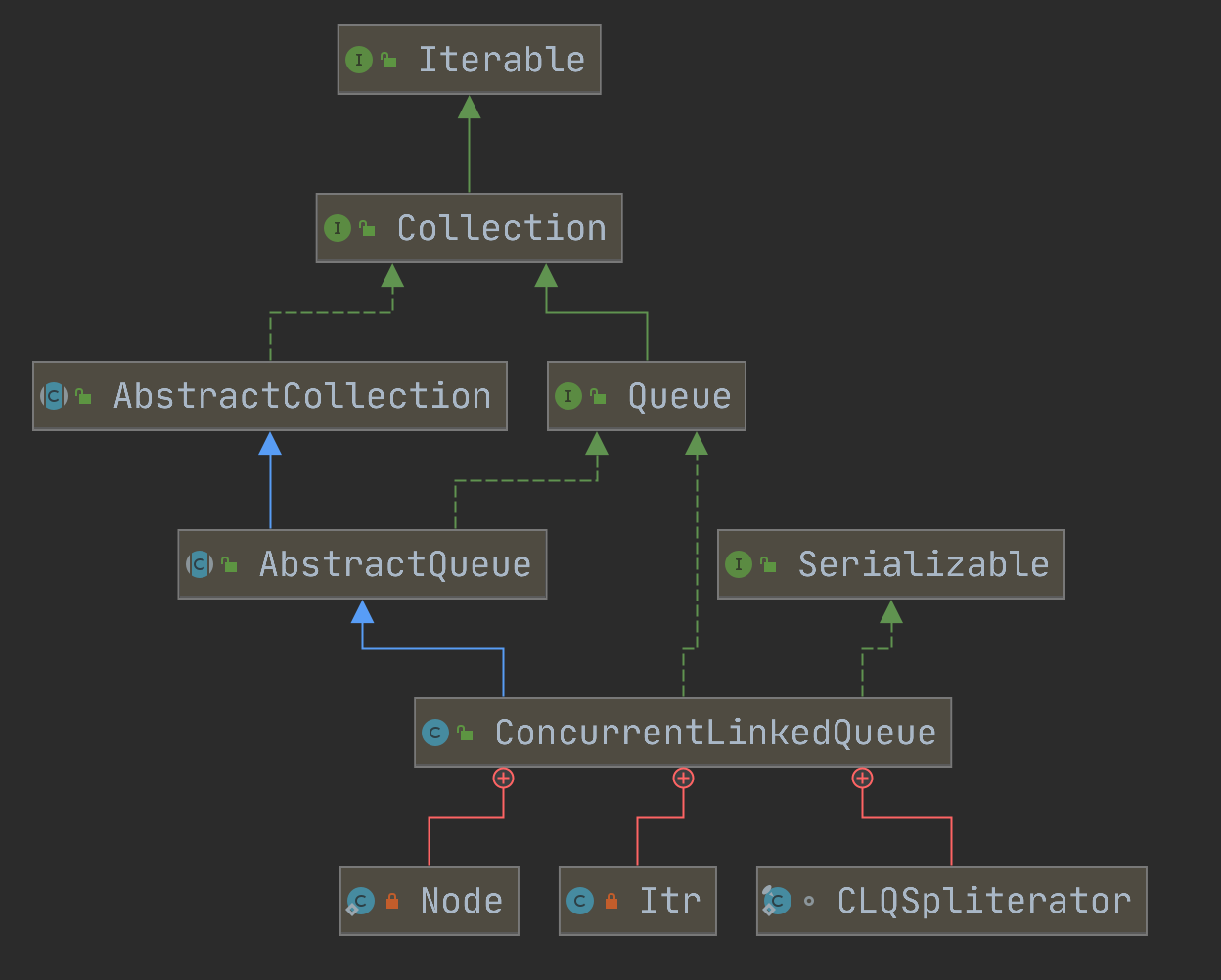#### 参数介绍

private static class Node<E> {        // 节点中的元素    volatile E item;    // 下一个节点    volatile Node<E> next;    Node(E item) {        UNSAFE.putObject(this, itemOffset, item);    }    // CAS 的方式设置节点元素    boolean casItem(E cmp, E val) {        return UNSAFE.compareAndSwapObject(this, itemOffset, cmp, val);    }    // 设置下一个节点    void lazySetNext(Node<E> val) {        UNSAFE.putOrderedObject(this, nextOffset, val);    }    // CAS 的方式设置下一个节点    boolean casNext(Node<E> cmp, Node<E> val) {        return UNSAFE.compareAndSwapObject(this, nextOffset, cmp, val);    }    // 省略 ……}

public class ConcurrentLinkedQueue<E> extends AbstractQueue<E>        implements Queue<E>, java.io.Serializable {            // 其他省略    // 头结点    private transient volatile Node<E> head;          // 尾节点    private transient volatile Node<E> tail;}

#### 构造函数

public ConcurrentLinkedQueue() {    head = tail = new Node<E>(null);}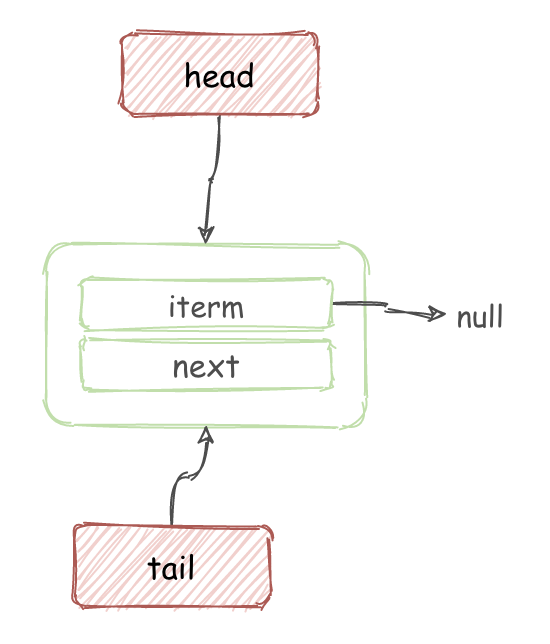#### 添加元素

public boolean add(E e) {    return offer(e);}public boolean offer(E e) {        // 验证是否为空    checkNotNull(e);    // 创建节点    final Node<E> newNode = new Node<E>(e);    // 循环入队列    // t 是当前尾节点，p 初始为 t    for (Node<E> t = tail, p = t;;) {        // q 为尾节点的下一个节点        Node<E> q = p.next;        if (q == null) {            // 为空，说明后面没有节点，则 CAS 设置尾节点            if (p.casNext(null, newNode)) {                // 此时 p.next 是 newNode                // 如果 p ！= t 说明有并发                if (p != t)                     // 其他线程已经更新了 tail                    // q = p.next 所以 q == null 不正确了                    // q 取到了 t.next                    // 此时将 tail 更新为 新节点                    casTail(t, newNode);  // Failure is OK.                return true;            }            // Lost CAS race to another thread; re-read next        }        // 多线程情况下， poll ，操作移除元素，可能会导致 p == q         // 此时要重新查找        else if (p == q)            //             p = (t != (t = tail)) ? t : head;        else            // 检查 tail 并更新            p = (p != t && t != (t = tail)) ? t : q;    }}

• 单线程情况下：

1. 当执行到 Node<E> q = p.next; 时，当前情况如图所示：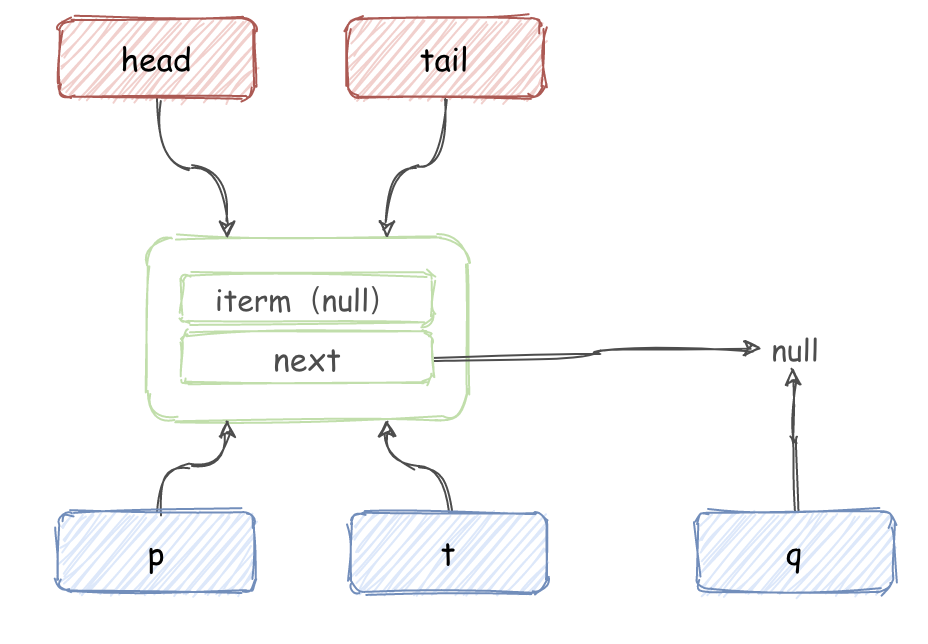1. 判断 q == null，满足条件，此时便会执行 p.casNext(null, newNode) 使用 CAS 设置 p.next。

2. 设置成功之后，p == t 没有变动，所以程序退出。

• 多线程情况下：

1. 当执行到 Node<E> q = p.next; 时，当前情况如图所示：1. 多个线程执行 p.casNext(null, newNode) 使用 CAS 设置 p.next。

2. A 线程 CAS 设置成功：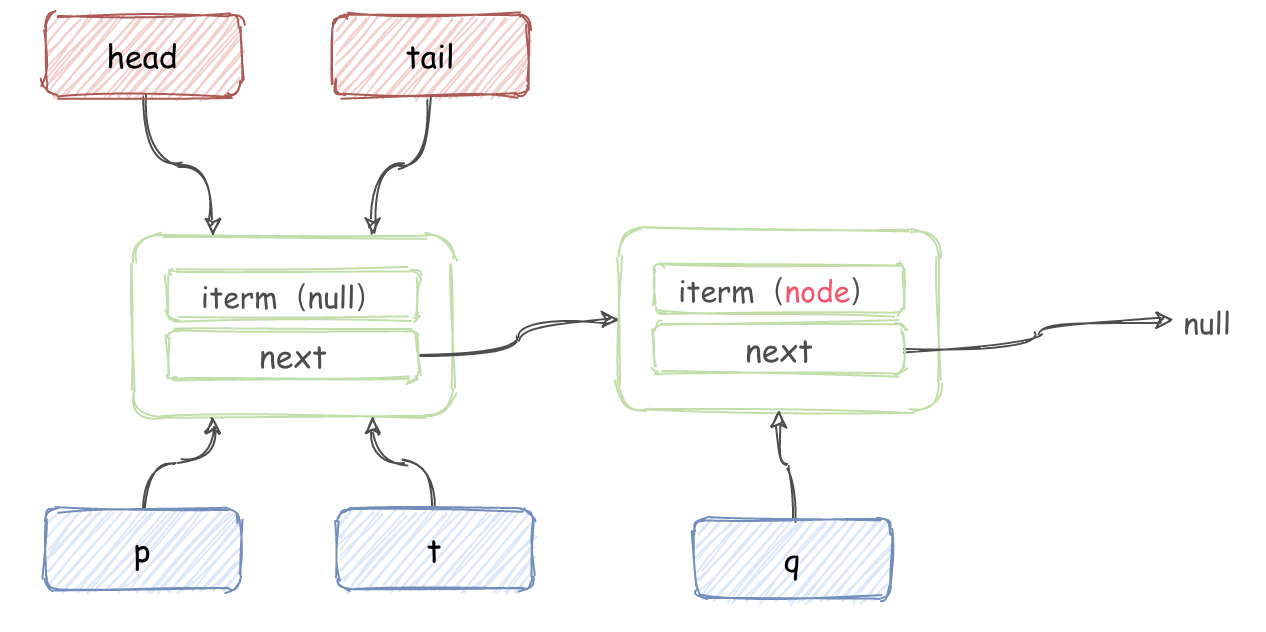1. B 线程 CAS 执行失败， 重新循环，会执行到 p = (p != t && t != (t = tail)) ? t : q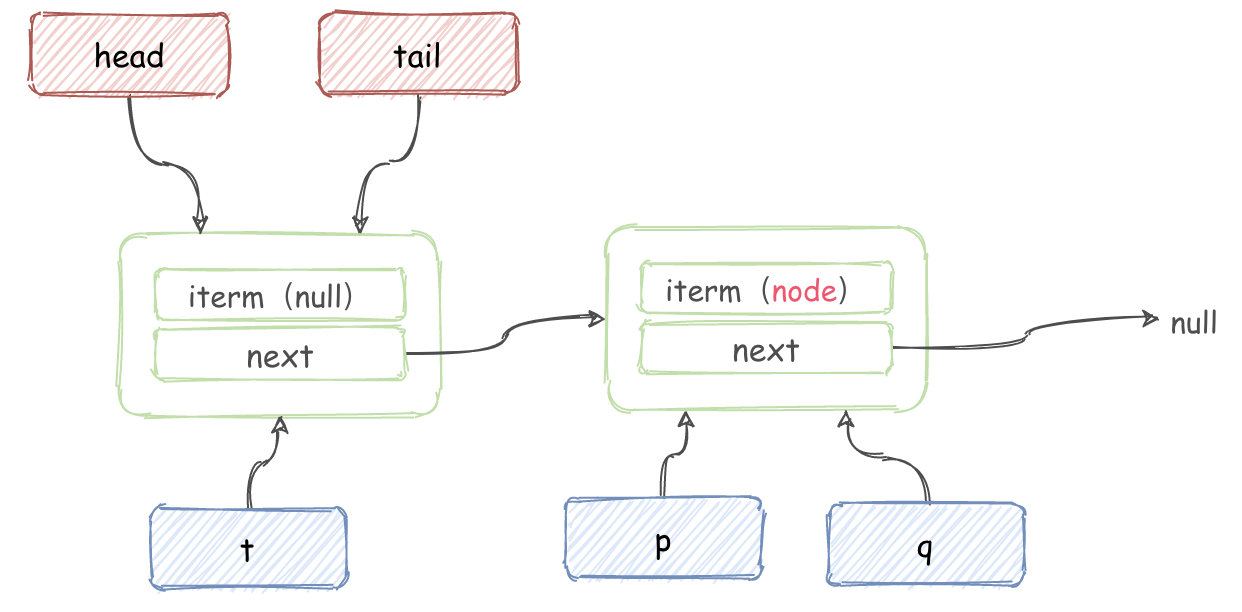1. 再次循环就可以成功设置上了。

#### 获取元素

public E poll() {    restartFromHead:    // 无限循环    for (;;) {        for (Node<E> h = head, p = h, q;;) {            // 头结点的 iterm            E item = p.item;            // 当前节点如果不为 null CAS 设置为 null            if (item != null && p.casItem(item, null)) {                // CAS 成功 则标记移除                if (p != h) // hop two nodes at a time                    updateHead(h, ((q = p.next) != null) ? q : p);                return item;            }            // 当前队列未空 返回 null            else if ((q = p.next) == null) {                updateHead(h, p);                return null;            }            // 自引用了， 重新进行循环            else if (p == q)                continue restartFromHead;            else                p = q;        }    }}

1. 在执行内层循环时，如果队列为空：E item = p.item; 此时，iterm 为 null，会 updateHead(h, p) 并返回 null。

2. 假设同时有并发插入操作，添加了一个元素，此时如图所示：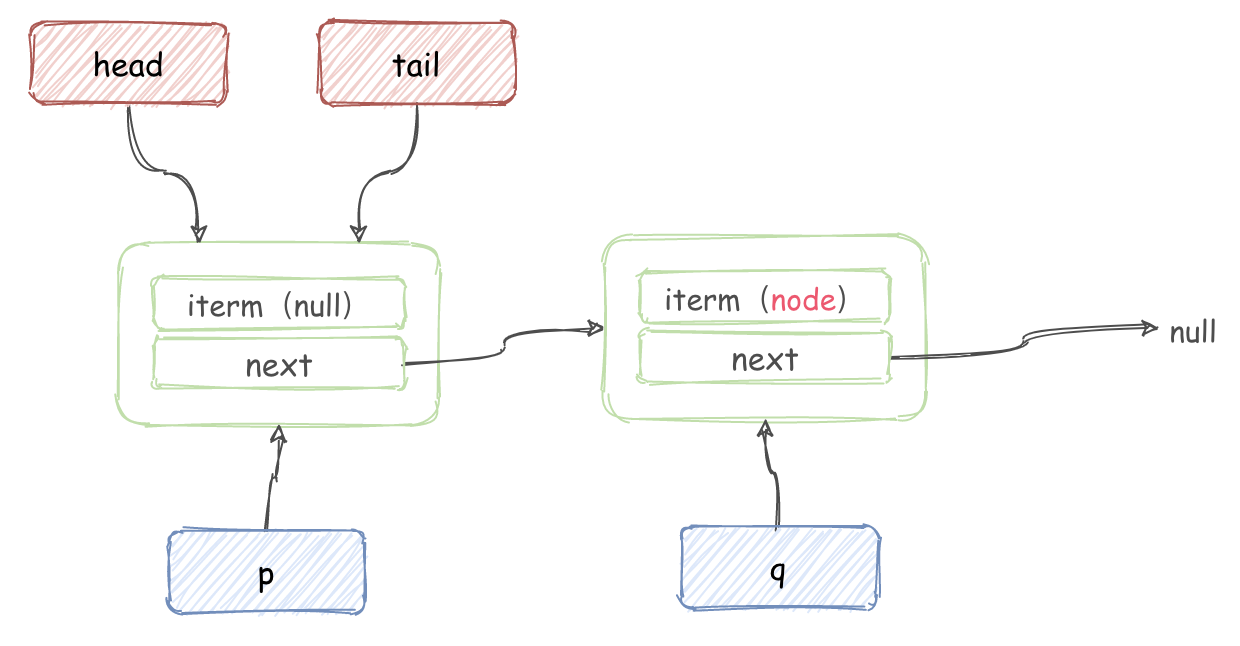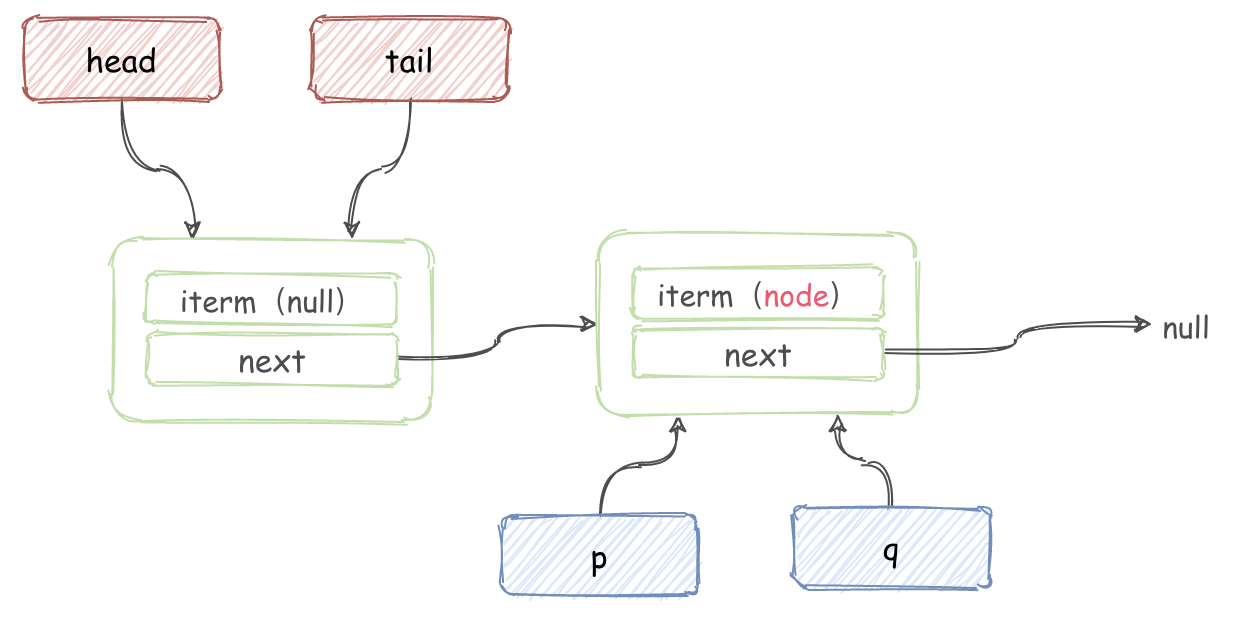1. 继续循环获取 item，并执行 p.casItem(item, null) ， 然后判断 p != h，更新 head 并返回 item。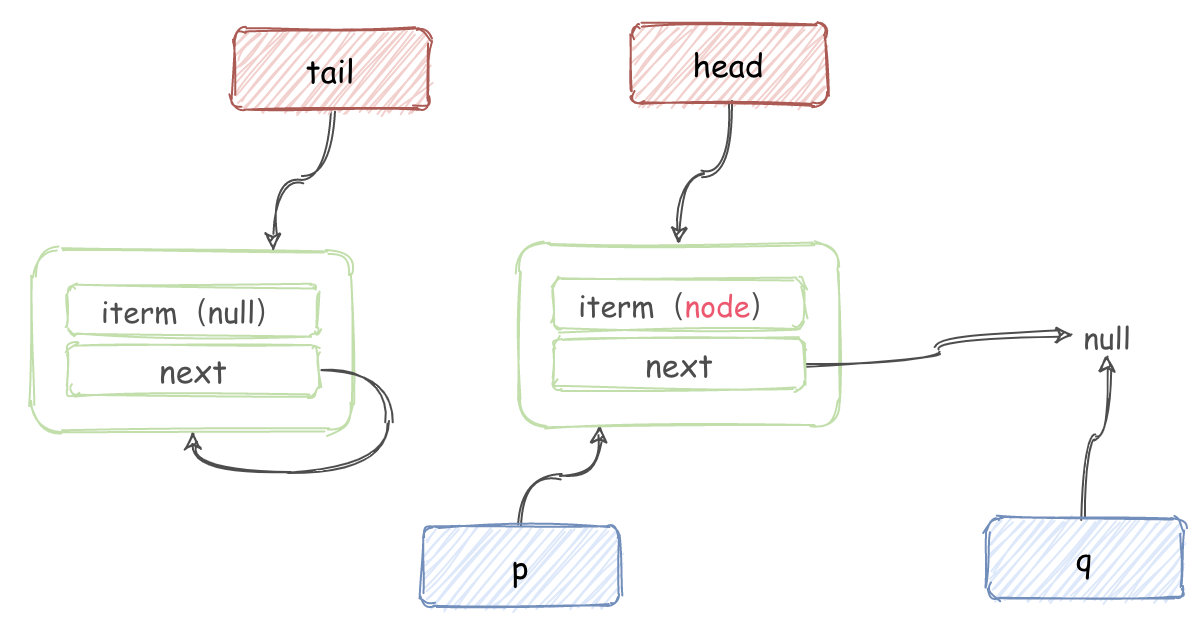#### size 操作

public int size() {    int count = 0;    for (Node<E> p = first(); p != null; p = succ(p))        if (p.item != null)            // Collection.size() spec says to max out            if (++count == Integer.MAX_VALUE)                break;    return count;}

CAS 没有加锁，所以 size 是不准确的。并且 size 会遍历一遍列表，比较耗费性能。

### 总结## 评论